# Visualizing Scientific Replication with scifigure

#### 2019-03-05

scifigure contains simple utilites for generating visual representations of scientific reproduction and replication efforts. This package is a companion to an article (Patil, Peng, and Leek 2016) that defines these efforts and explains why such visualizations are helpful. In this vignette, we will demonstrate how to use the functions in this package and recreate the main figure in (Patil, Peng, and Leek 2016).

## Basic usage

We have defined a general set of steps that comprise the conduct of a scientific study, and have compiled a set of icons to represent each step. Let us visualize these steps and icons for two studies using the scifigure package:

library(scifigure)
exps <- init_experiments(2)
sci_figure(exps)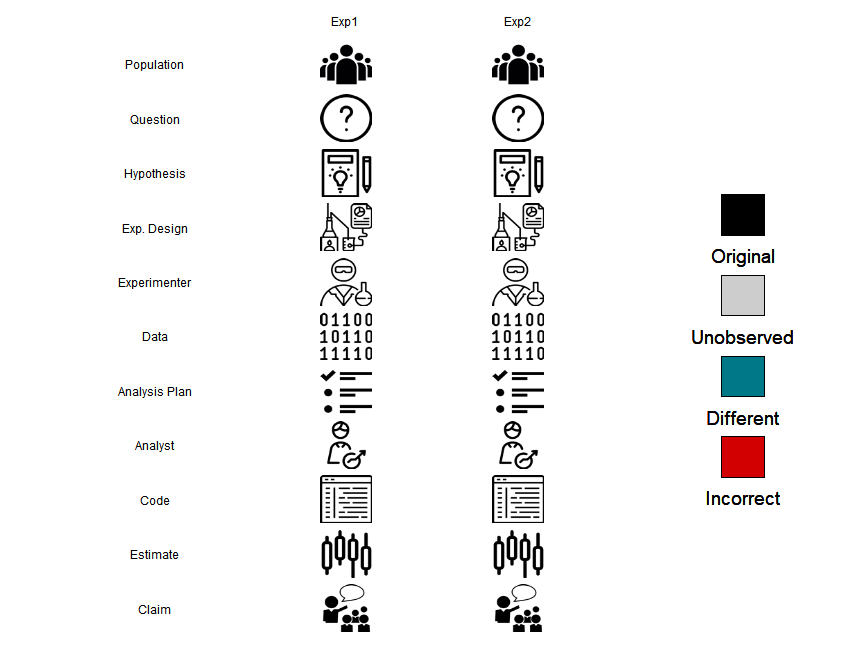To produce this figure, we first initialize a data frame using init_experiments, passing the parameter value 2 to indicate that the data frame should represent two studies (with default names for each experiment). We then pass this data frame to sci_figure, which renders the figure based on the contents of exps. Note that a legend is automatically generated, indicating that each step in each of the experiments is “observed”. We can modify the contents of exps to change change how elements of each experiment are displayed.

exps <- init_experiments(2, c("Brady et. al.", "Irving et. al."))
exps["analysis_plan", 1] <- "unobserved"
exps[c("experimenter", "analyst", "estimate"), 2] <- "different"
sci_figure(exps, hide_stages = c("population", "hypothesis"))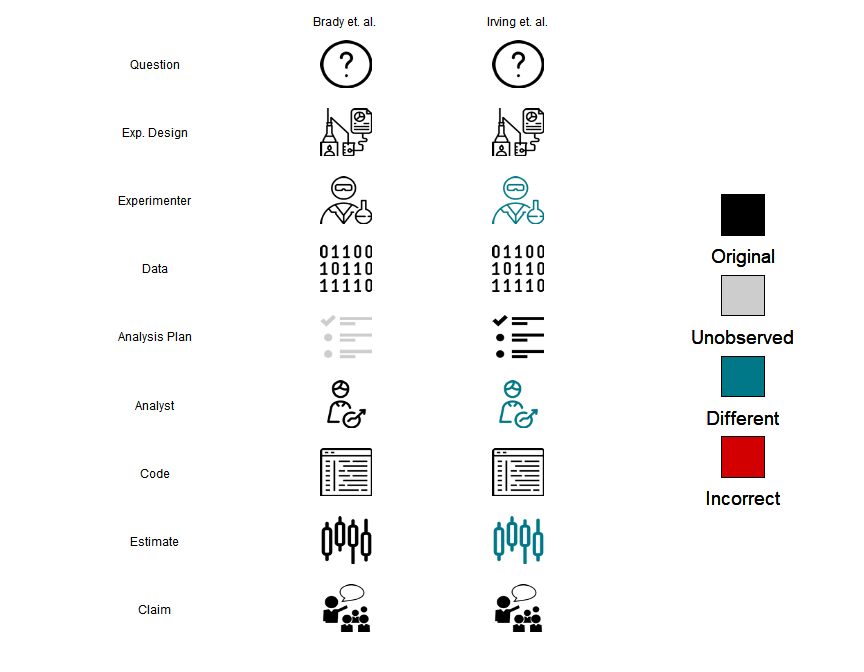Above we first specified experiment names for each experiment when we initialized the data frame. These names will be the column headers in the figure. Next, we modified some of the elements of the data frame: the analysis plan in the first study is missing, and the experimenter, analyst, and estimate in the second study differed from the first. Finally, when generating the visualization we hide the population and hypothesis stages.

To emphasize the differences between the studies, the difference mode can be used via the diff flag:

sci_figure(exps, hide_stages = c("population", "hypothesis"), diff = TRUE)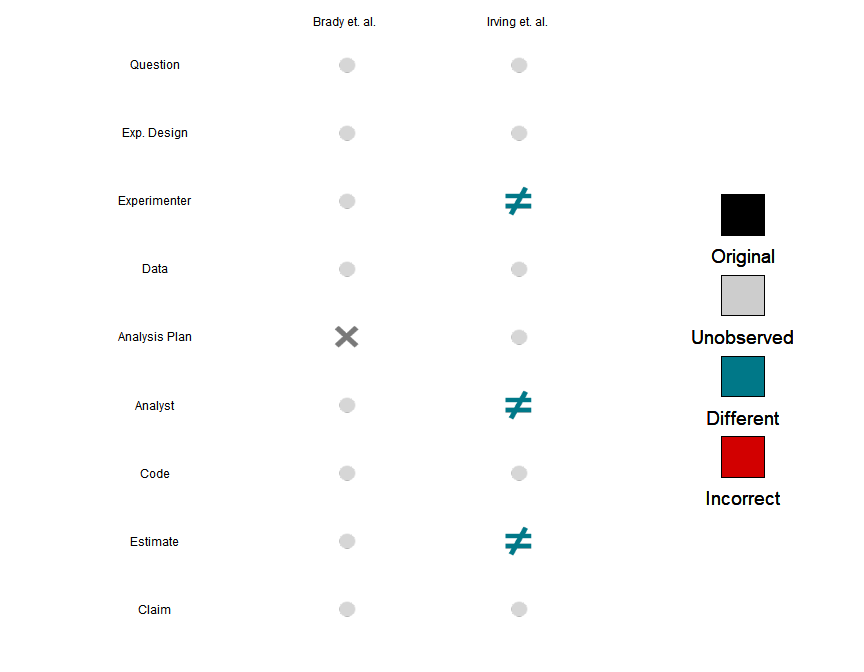The difference mode uses a set of four symbols that were chosen to be semantically close to the scenarios that they are encoding, i.e. “unobserved” is “$$\times$$”, “different” is “$$\neq$$”, and “incorrect” is “$$!$$”. The least informative scenario in which both studies are the same is de-emphasized.

We have also included convenience wrapper functions to generate visualizations of our definitions of reproducibility and replicability given in (Patil, Peng, and Leek 2016):

reproduce_figure()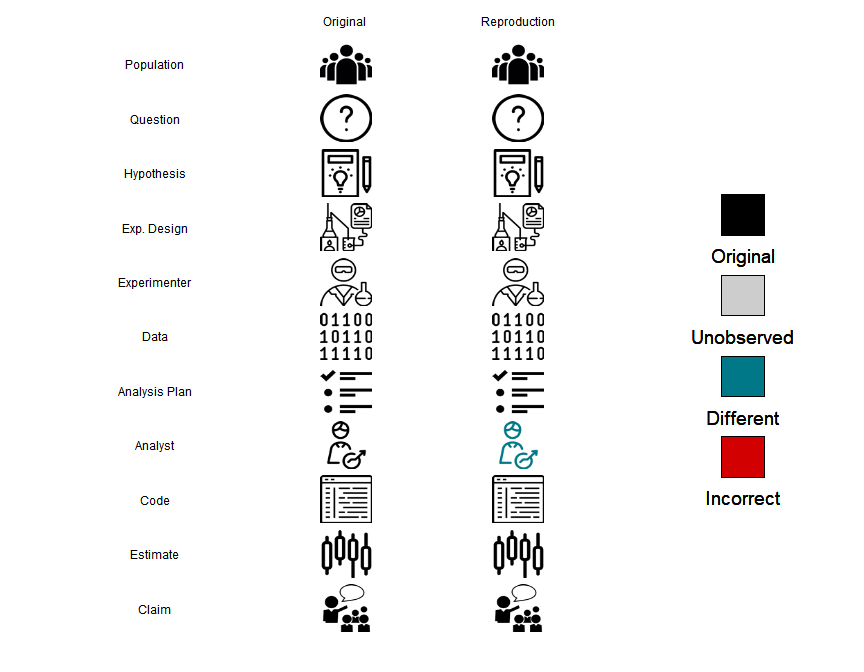replicate_figure()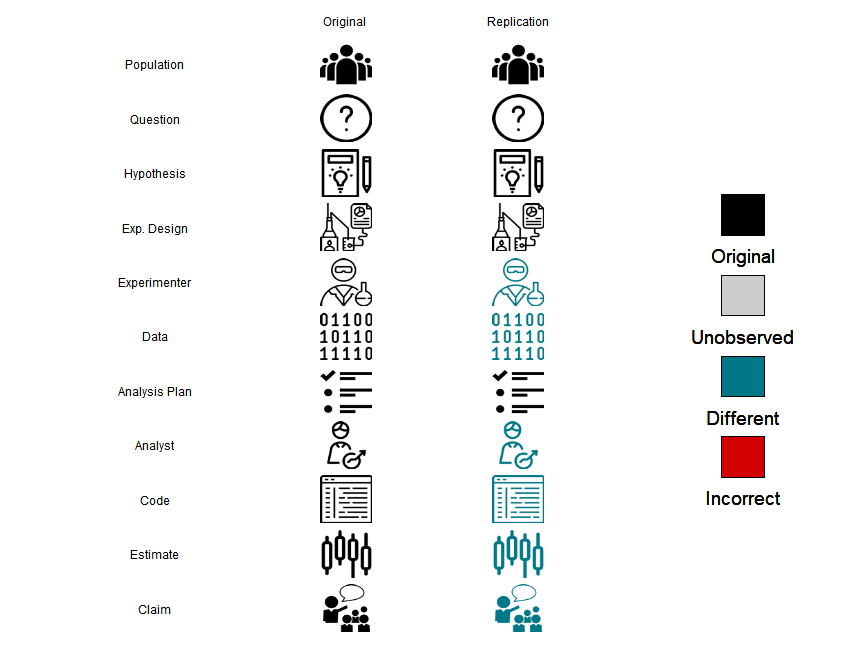## Recreating Figure 1

The following code snippet recreates the columns in Figure 1 of (Patil, Peng, and Leek 2016):

exps <- init_experiments(9, c("Original", "Reproducible", "Orignal", "Replicable", "Begley", "Payne et. al.", "Vianello (OSF)", "Potti", "Baggerly & Coombs"))
exps["analyst", 2] <- "different" # Reproducible
exps[c("experimenter", "data", "analyst", "code", "estimate", "claim"), 4] <- "different" # Replicable
exps[c("population", "hypothesis", "experimental_design", "experimenter", "data", "analysis_plan", "analyst", "code", "estimate"), 5] <- "unobserved" # Begley
exps[c("population", "experimenter", "data", "analyst", "code", "estimate", "claim"), 7] <- "different" # Vianello (OSF)
exps[c("data", "code"), 8] <- "incorrect" # Potti
exps[c("data", "code"), 9] <- "different" # Baggerly & Coombes

sci_figure(exps)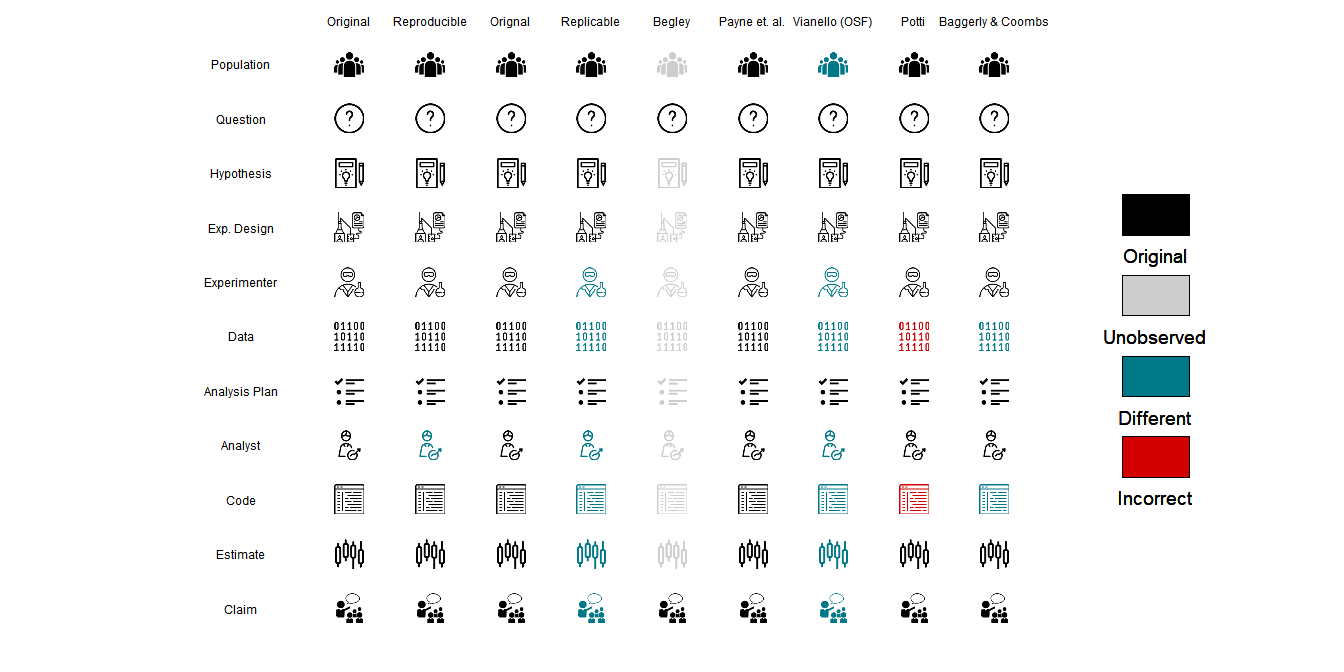Here is the same figure using the difference mode, emphasizing what is different between the studies:

sci_figure(exps, diff = TRUE)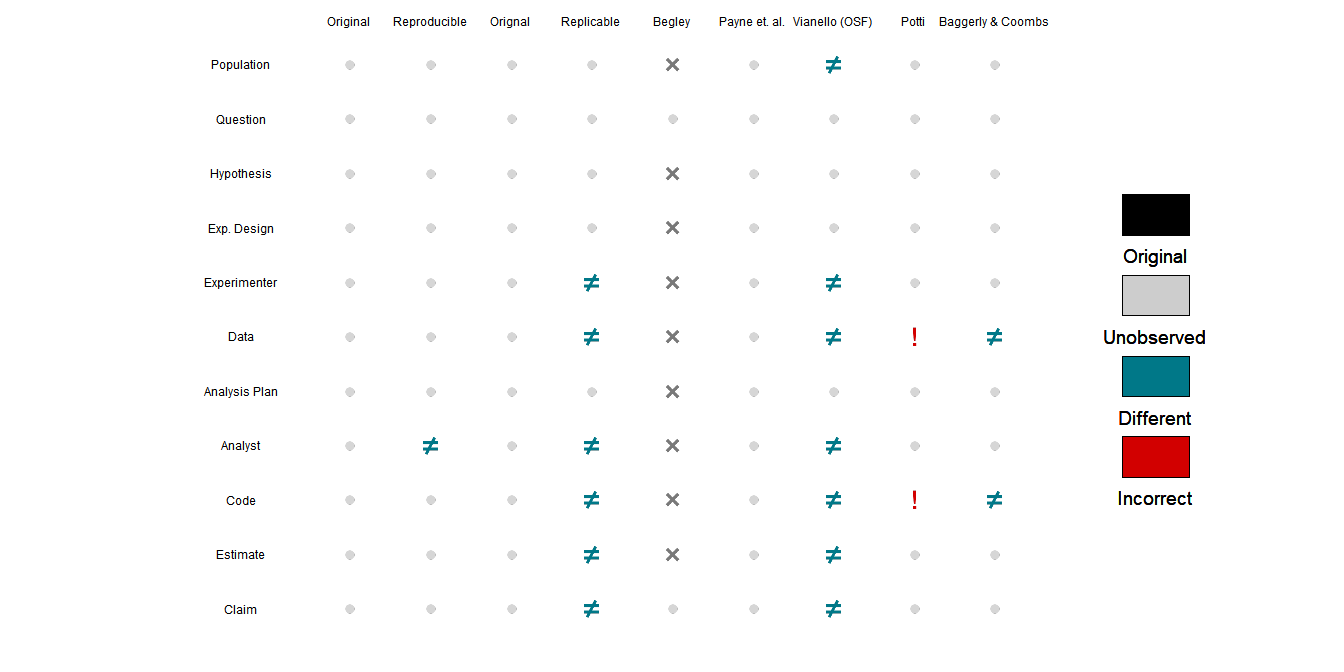## Using Custom Icons

Users may specificy custom icons for a scientific study protocol of their choosing using the custom_icons option for sci_figure. These icons must be read into R and provided as a list in the proper nomenclature to the function. There must be one icon for each of the states (observed, unobserved, different, incorrect), and the list names must be of the format (stage name)_(state) (see below). Default colors for the four states can be found in the documentation of the sci_figure function, or custom legend colors can be specified with the col option. We recommend icons be 75 by 75 pixels or smaller. Below we go through an example of specifying a custom set of four icons. These icons are being loaded from inst/extdata, but could be read from any file folder.

library(png)
## Warning: package 'png' was built under R version 3.4.4
questionnaire_observed <- readPNG(system.file("extdata", "questionnaire_observed.png", package = "scifigure"), native = T)
questionnaire_different <- readPNG(system.file("extdata", "questionnaire_different.png", package = "scifigure"), native = T)
questionnaire_incorrect <- readPNG(system.file("extdata", "questionnaire_incorrect.png", package = "scifigure"), native = T)
questionnaire_unobserved <- readPNG(system.file("extdata", "questionnaire_unobserved.png", package = "scifigure"), native = T)

measurement_observed <- readPNG(system.file("extdata", "measurement_observed.png", package = "scifigure"), native = T)
measurement_different <- readPNG(system.file("extdata", "measurement_different.png", package = "scifigure"), native = T)
measurement_incorrect <- readPNG(system.file("extdata", "measurement_incorrect.png", package = "scifigure"), native = T)
measurement_unobserved <- readPNG(system.file("extdata", "measurement_unobserved.png", package = "scifigure"), native = T)

analysis_observed <- readPNG(system.file("extdata", "analysis_observed.png", package = "scifigure"), native = T)
analysis_different <- readPNG(system.file("extdata", "analysis_different.png", package = "scifigure"), native = T)
analysis_incorrect <- readPNG(system.file("extdata", "analysis_incorrect.png", package = "scifigure"), native = T)
analysis_unobserved <- readPNG(system.file("extdata", "analysis_unobserved.png", package = "scifigure"), native = T)

result_observed <- readPNG(system.file("extdata", "result_observed.png", package = "scifigure"), native = T)
result_different <- readPNG(system.file("extdata", "result_different.png", package = "scifigure"), native = T)
result_incorrect <- readPNG(system.file("extdata", "result_incorrect.png", package = "scifigure"), native = T)
result_unobserved <- readPNG(system.file("extdata", "result_unobserved.png", package = "scifigure"), native = T)

icon_list <- list("questionnaire_observed"=questionnaire_observed, "questionnaire_different"=questionnaire_different,
"questionnaire_incorrect"=questionnaire_incorrect, "questionnaire_unobserved"=questionnaire_unobserved,
"measurement_observed"=measurement_observed, "measurement_different"=measurement_different,
"measurement_incorrect"=measurement_incorrect, "measurement_unobserved"=measurement_unobserved,
"analysis_observed"=analysis_observed, "analysis_different"=analysis_different,
"analysis_incorrect"=analysis_incorrect, "analysis_unobserved"=analysis_unobserved,
"result_observed"=result_observed, "result_different"=result_different,
"result_incorrect"=result_incorrect, "result_unobserved"=result_unobserved)

stage_names <- c("questionnaire", "measurement", "analysis", "result")
stage_names2 <- c("Questionnaire", "Measurement", "Analysis", "Result")

exps <- init_experiments(nexp = 3, stage_names = stage_names)
sci_figure(exps, custom_icons = icon_list, stage_names = stage_names2)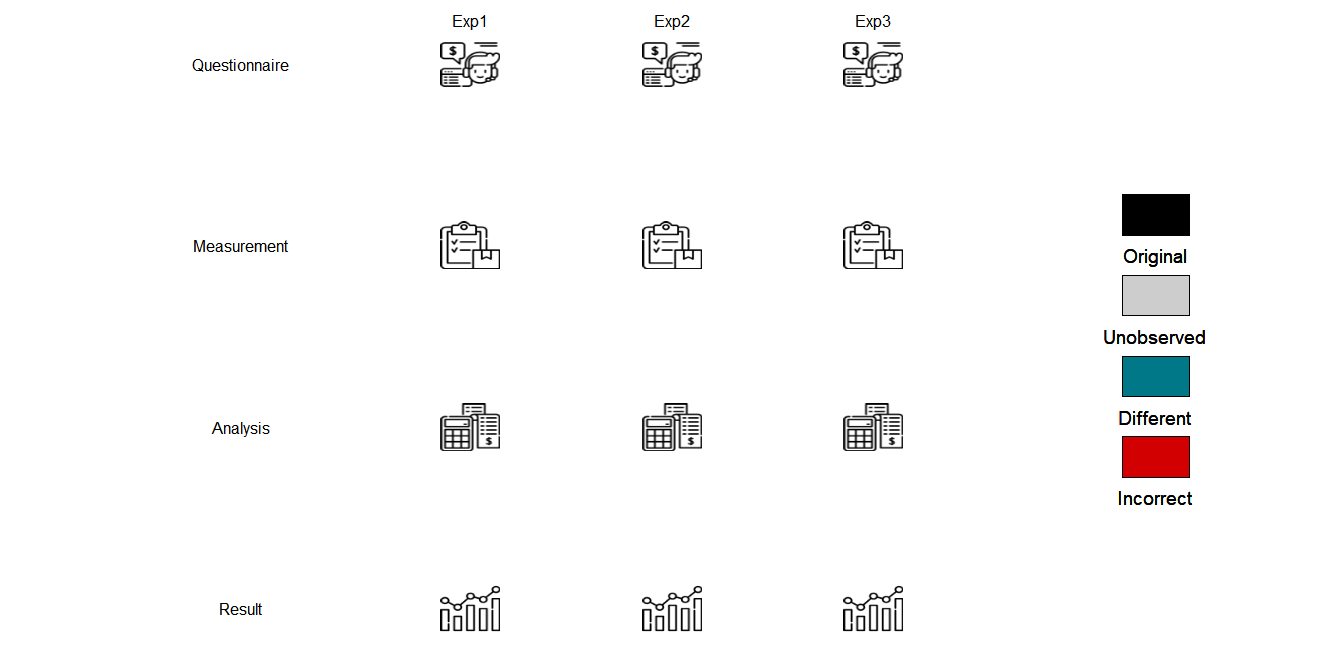exps["analysis", "Exp1"] <- "different"
exps["questionnaire", "Exp2"] <- "incorrect"
exps["result", c("Exp2", "Exp3")] <- "unobserved"
sci_figure(exps, custom_icons = icon_list, stage_names = stage_names2)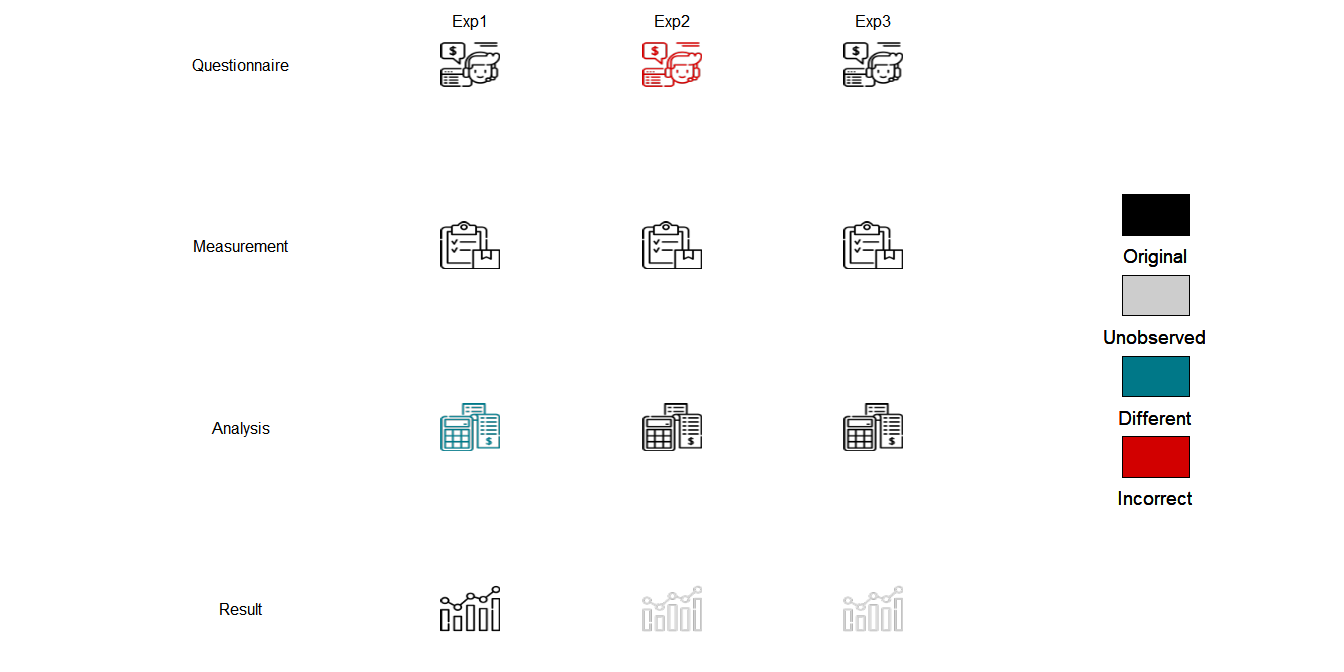Another, simpler option would be to use diff = T mode and have ambiguous icons represent the custom stages you wish to display.